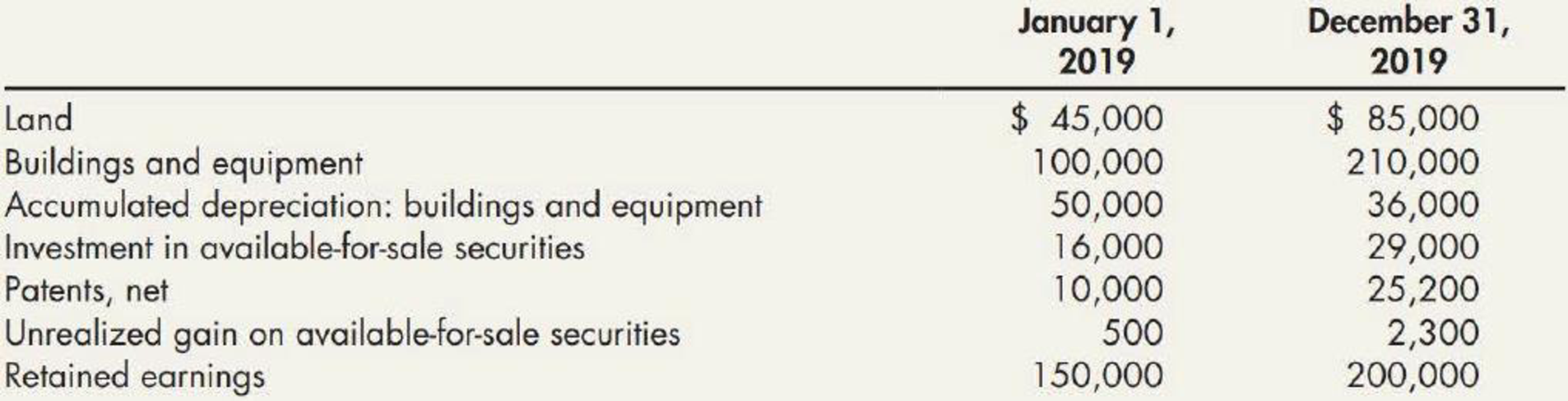Chapter 21, Problem 17E### Intermediate Accounting: Reporting...

3rd Edition
James M. Wahlen + 2 others
ISBN: 9781337788281

#### Solutions

Chapter
Section### Intermediate Accounting: Reporting...

3rd Edition
James M. Wahlen + 2 others
ISBN: 9781337788281
Textbook Problem
1 views

# Investing Activities and Depreciable Assets Verlando Company had the following account balances and information available for 2019:During 2019, Verlando recorded the following transactions affecting these accounts: a. Land with a carrying value of $35,000 was sold at a loss of$6,000. b. Land and equipment were purchased with cash during the period. c. Equipment with an original cost of $20,000 that had a book value of$4,000 was written off as obsolete. d. A building with an original cost of $60,000 and accumulated depreciation of$25,000 was sold at a $23,000 gain. e. Depreciation expense and amortization expense were recorded. f. Net income for the year was$60,000. g. A patent was acquired during the year in exchange for 1,200 shares of common stock with a par value of $1 per share and a market value of$26 per share. h. Additional marketable securities wefe purchased during the year. i. Verlando Company has no notes payable in the liabilities section of its balance sheet. Required: 1. Next Level Assuming that Verlando uses the indirect method to determine operating cash flows, what is the amount of depreciation expense and amortization expense that would be added back to net income: 2. Prepare the investing activities section of the statement of cash flows for the year ended December 31, 2019. 3. Prepare the disclosure for significant noncash transactions for the statement of cash flows for the year ended December 31, 2019.

1.

To determine

Calculate the amount of depreciation expense and amortization expense of V Company for the year 2019.

Explanation

Depreciation expense: Depreciation expense is non-cash expenses, which is recorded on the income statement reflecting the consumption of economic benefits of long-term asset on account of its wear and tear or obsolesce.

Calculate the amount of depreciation expense.

Depreciation expense=Ending balance{Beginning balance+Accumulated depreciation removed due to write off+Accumulated depreciation removed due to sale  }

Depreciation expense =($36,000$50,000+$16,000+$25,000)=$27,000 Therefore, the amount of depreciation expense is$27,000.

Amortization: Amortization is a process in which the cost of intangible assets is spread equally over the limited or definite useful life of asset

2.

To determine

Report the investing activities section in the cash flow statement of V Company for the year ended 2019.

3.

To determine

Report the disclosure of non-cash transaction in cash flow statement for the year ended 2019.

### Still sussing out bartleby?

Check out a sample textbook solution.

See a sample solution

#### The Solution to Your Study Problems

Bartleby provides explanations to thousands of textbook problems written by our experts, many with advanced degrees!

Get Started